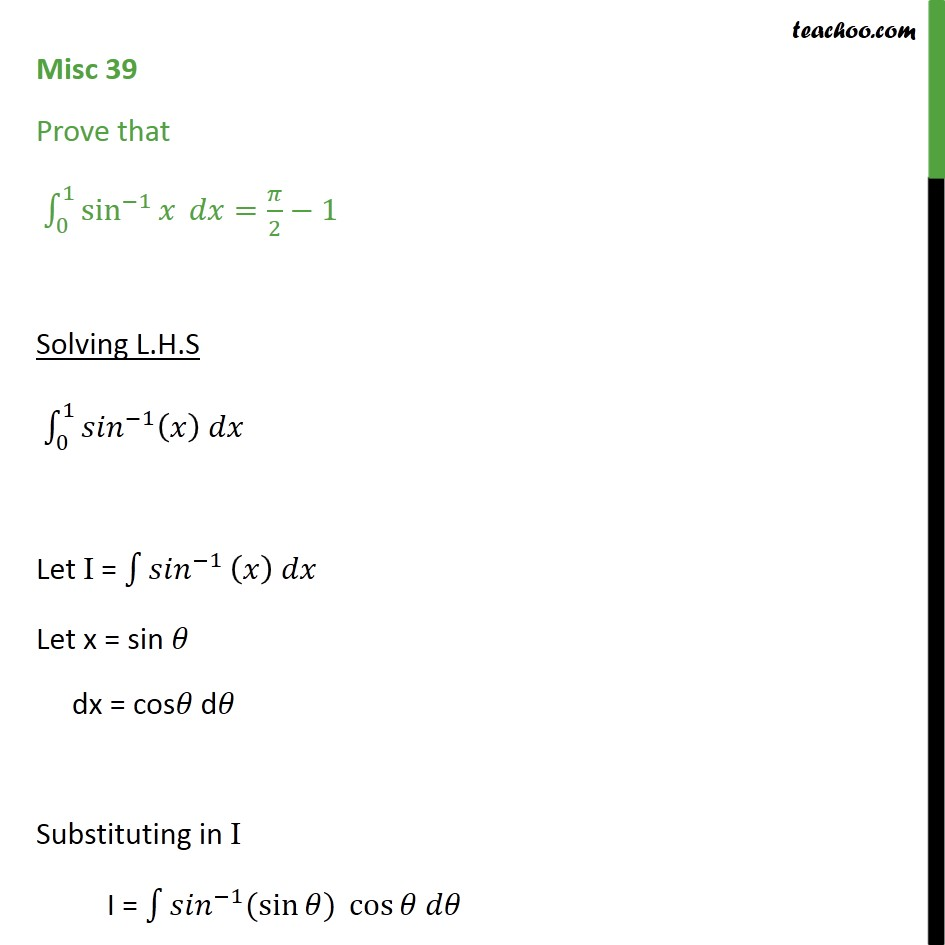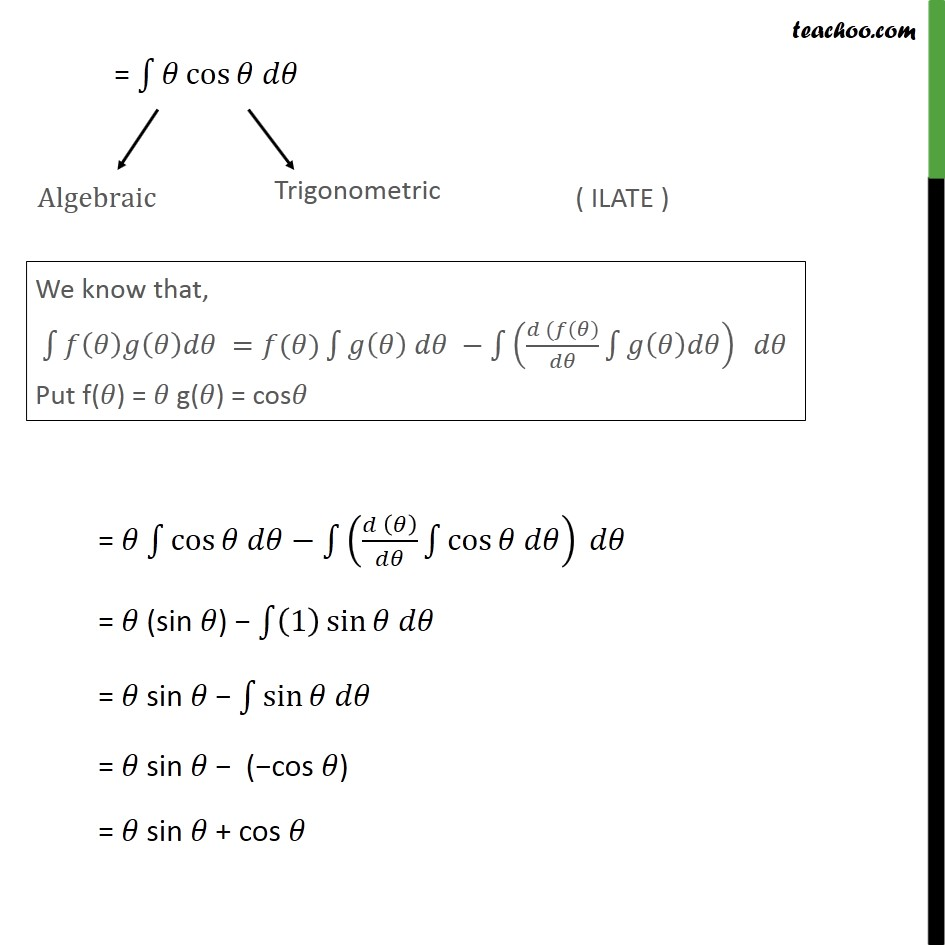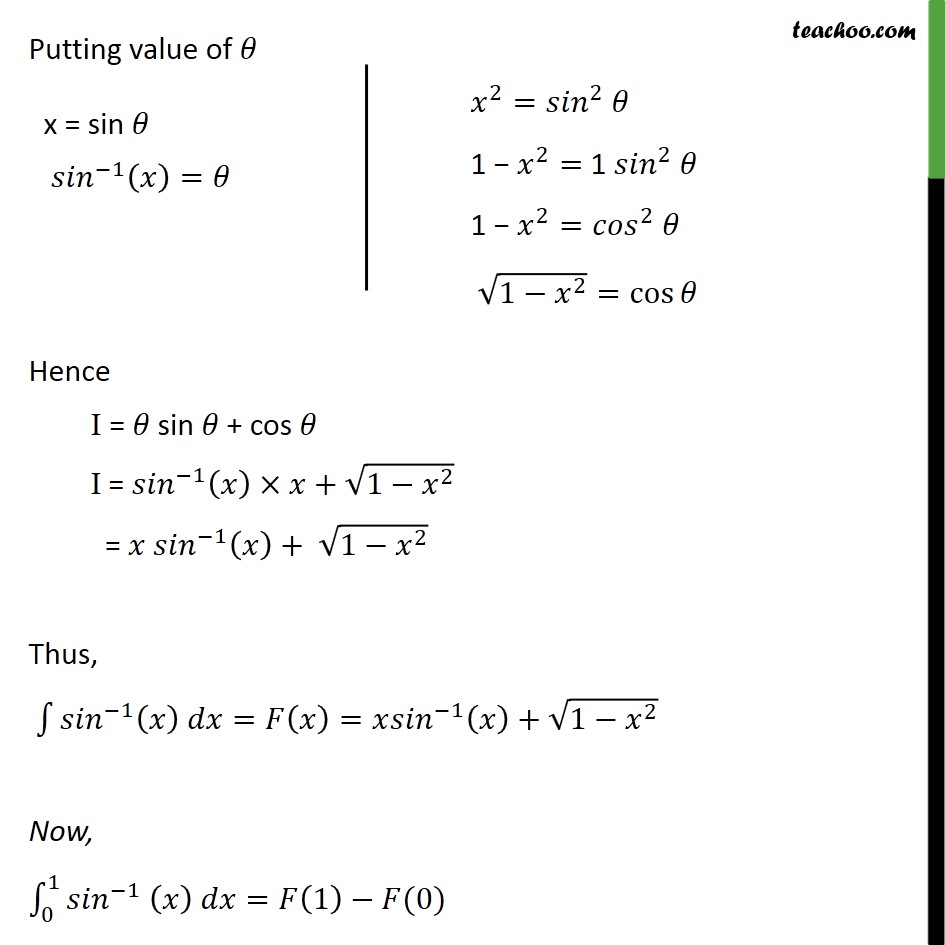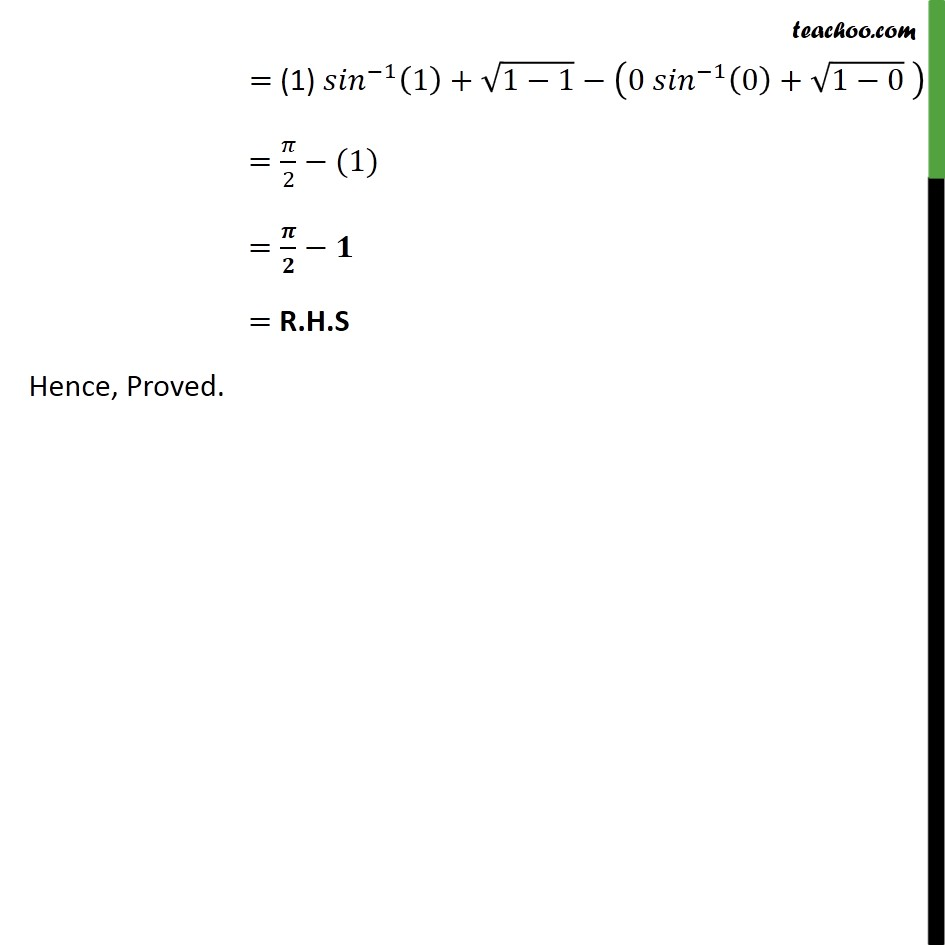Integration Full Chapter Explained - Integration Class 12 - Everything you need1. Chapter 7 Class 12 Integrals
2. Concept wise
3. Definite Integration - By Formulae

Transcript

Misc 39 Prove that 0 1 sin 1 = 2 1 Solving L.H.S 0 1 1 Let I = 1 Let x = sin dx = cos d Substituting in I I = 1 ( sin ) cos = cos = cos cos = (sin ) 1 sin = sin sin = sin ( cos ) = sin + cos Putting value of Hence I = sin + cos I = 1 + 1 2 = 1 + 1 2 Thus, 1 = = 1 + 1 2 Now, 0 1 1 = 1 (0) = (1) 1 1 + 1 1 0 1 0 + 1 0 = 2 (1) = = R.H.S Hence, Proved.

Definite Integration - By Formulae

About the AuthorDavneet Singh
Davneet Singh is a graduate from Indian Institute of Technology, Kanpur. He has been teaching from the past 9 years. He provides courses for Maths and Science at Teachoo.